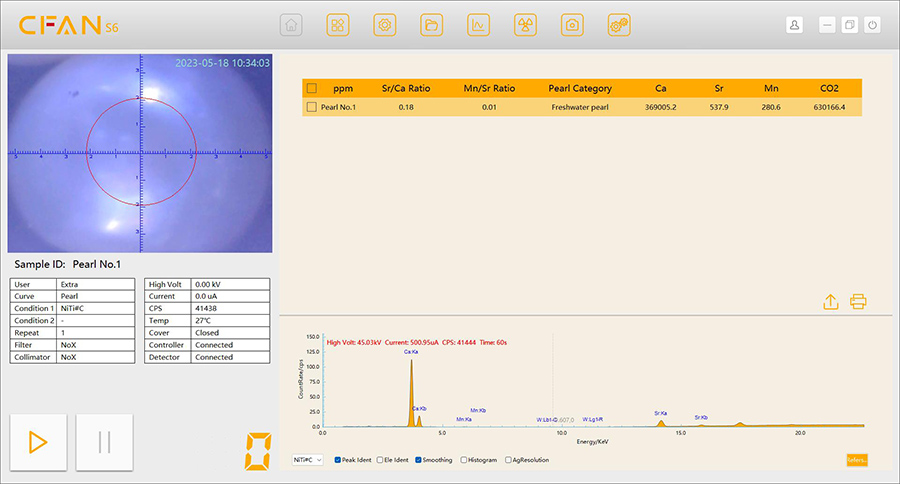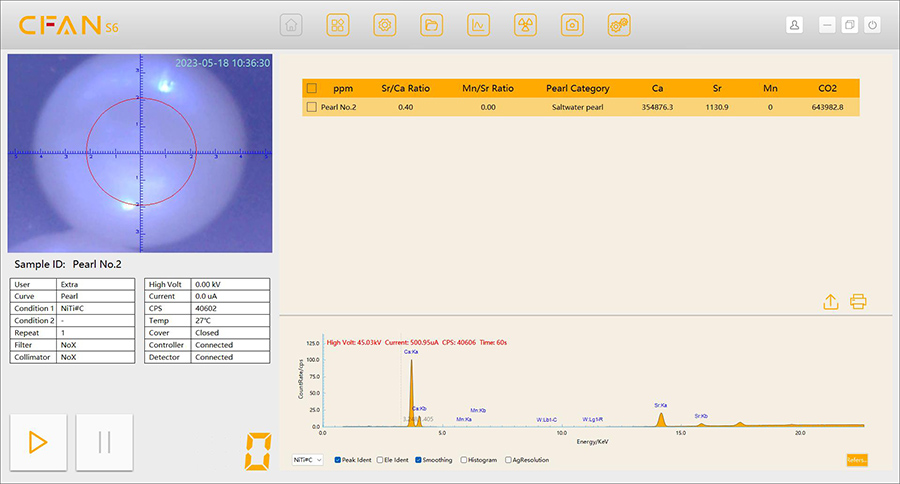EN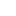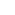Contact Us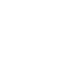Return

## XRF Secondary Programming - "Formula Editor"Introduction to Formula editor:

In 2022, CFAN launched its new product software "Smart FP", which provides a powerful software function that offers a wide range of operators and functions to help customers program their test results or test intensities for each element and display the results of the secondary programming in the software interface. It is convenient for customers to test special applications to display the results quickly and easily, without the need for additional manual calculation of markers. Customers can research various application scenarios on their own or build unique applications with the assistance of an application engineer. It can be used for a wide range of specific testing projects such as pearl type classification, stainless steel grade design, K-gold classification, ore powder and plastic RoHS classification, etc.

Operators in the formula editor include arithmetic operators (+,-,*,/) and logical operators(<,>,==,! =,<=,>=,&,||,()?a:b); functions including trigonometric functions (sin(x),cos(x),tan(x)), miscellaneous functions (pow(x,y),exp(x),sqrt(x),sign(x),abs(x)), variable parameter functions (sum (x1, x2...), avg (x1, x2...), min (x1, x2...), max (x1, x2...)), etc.

Below, we will use the classification of pearl types as an example to illustrate the operation of this function and demonstrate its effectiveness.

Identification of seawater and freshwater pearls:

The national standard GB/T 16552-2010 Jewellery and Jade Names describes that seawater pearls are relatively low in Mn and high in Sr, while freshwater pearls are relatively enriched in Mn and low in Sr. Using the obvious difference in the content of trace elements Sr and Mn between seawater and freshwater pearls, the strontium to calcium ratio (Sr/Ca), the manganese to calcium ratio (Mn/Ca) and the manganese to strontium ratio (Mn/Sr) of the main element Ca and the trace elements Sr and Mn were tested using X-ray fluorescence spectroscopy, and the relationship between the two or a combination of the three could be used to distinguish seawater pearls from freshwater pearls.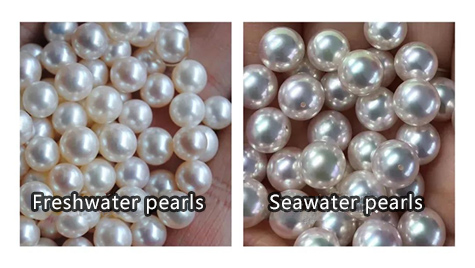XF software custom formula identification steps for seawater pearls and freshwater pearls: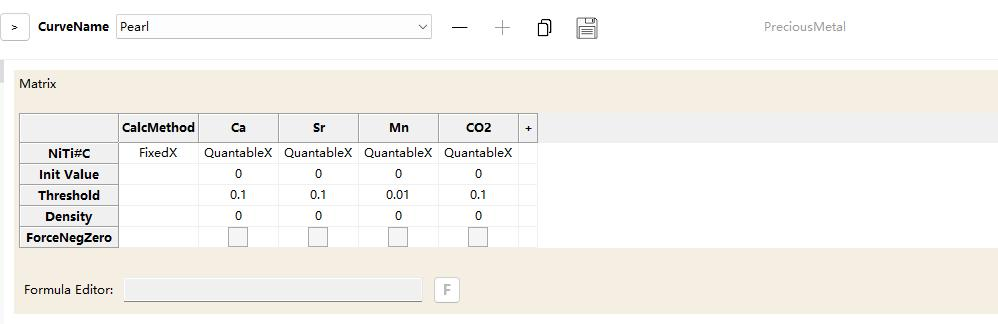1.Access the curve editing screen as shown below:

2.In the curve editing interface, check the formula editor button to pop up the formula list interface, as shown below: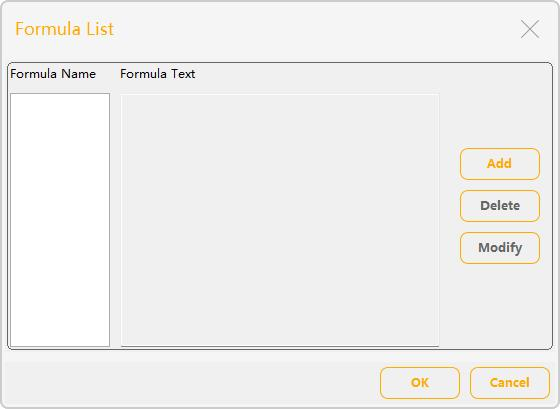3.Add the Strontium to calcium strength ratio formula

a.Click on the "Add" button in the formula list window to open the Define Formula window;

b.In the formula name, enter the name "Sr/Ca Ratio";

c.Check the "Elemental intensity" option for the elemental value type (Use intensity for Sr and Ca values);

d.Check the "Value" option for the type of result value, retaining 2 decimal places and not entering reporting units;

e.Enter the formula "Sr/Ca" in the formula text input box, as shown below: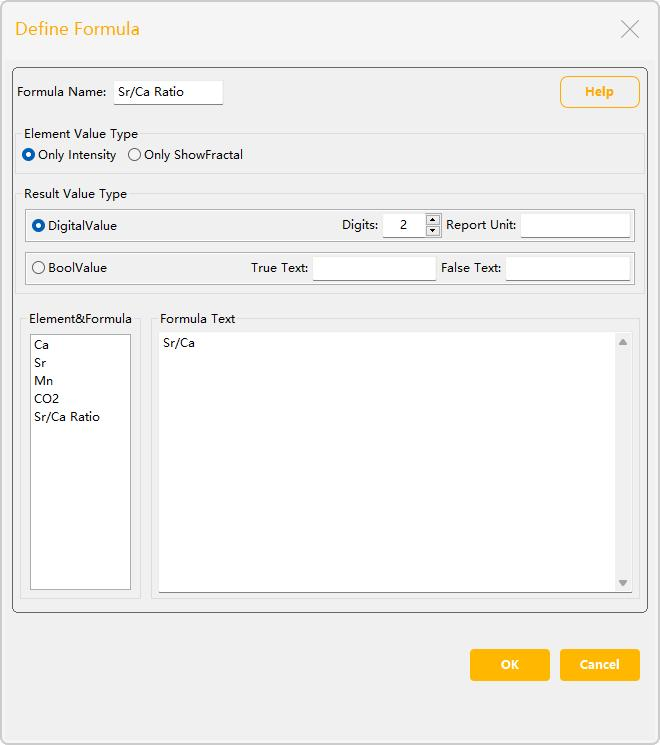f.Click on the "OK" button to add the current formula;

g.Once added, the list of formulas will show the name of the currently added formula, as shown below: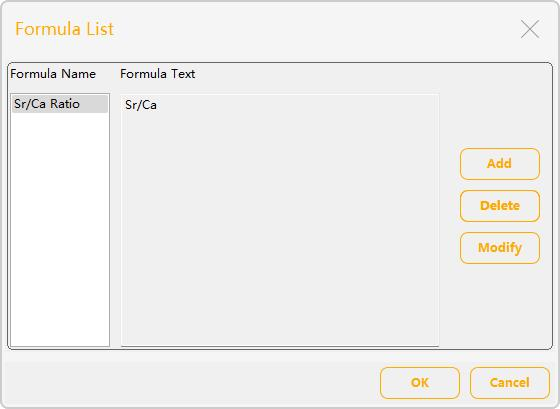4.Add Manganese to strontium strength ratio formula

a.Click on the "Add" button in the formula list window to open the Define Formula window;

b.In the formula name, enter the name "Mn/Sr Ratio";

c.The option "Elemental intensity" is selected for the elemental value type (Use intensity for the values of Mn and Sr);

d.Check the "Numeric" option for the type of result value, retaining 2 decimal places and not entering reporting units;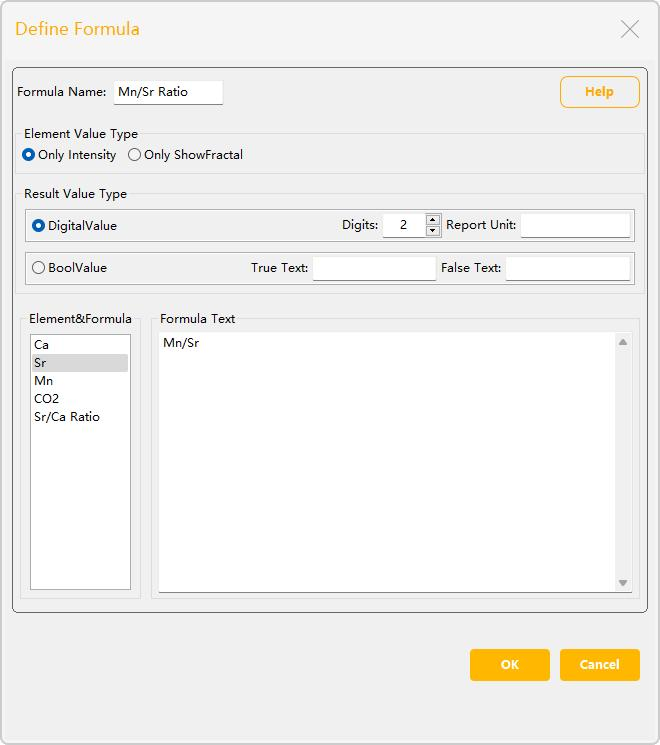e.Enter the formula "Mn/Sr" in the formula text input box, as shown below:

f.Click on the "OK" button to add the current formula;

g.Once added, the list of formulas will show the name of the currently added formula, as shown below: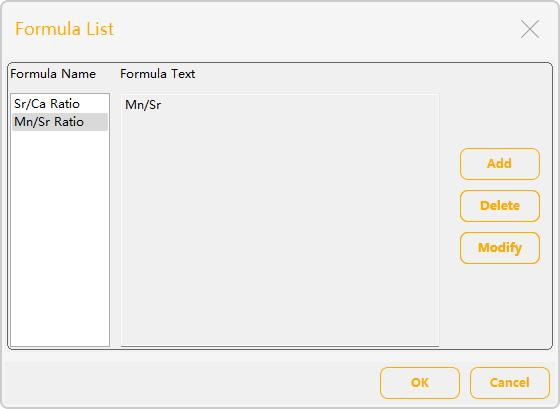5.Add formulas for identifying pearl categories based on the Sr/Ca and Mn/Sr strength formula

a.Click on the "Add" button in the formula list window to open the Define Formula window;

b.In the formula name, enter the name "Pearl Category";

c.Check the "Element intensity" option for the element value type;

d.Check the 'Judgement value' option for the result value type and enter 'Seawater pearl' for the True Text text and 'Freshwater pearl' for the False Text text.

e.Enter the formula "(Sr/Ca Ratio>0.2)&&(Mn/Sr Ratio<0.01)" in the formula text input box. That is, when the strontium to calcium ratio is >0.2 and the manganese to strontium ratio is <0.01, the pearl is judged to be a saltwater pearl, otherwise it is a freshwater pearl, as shown below: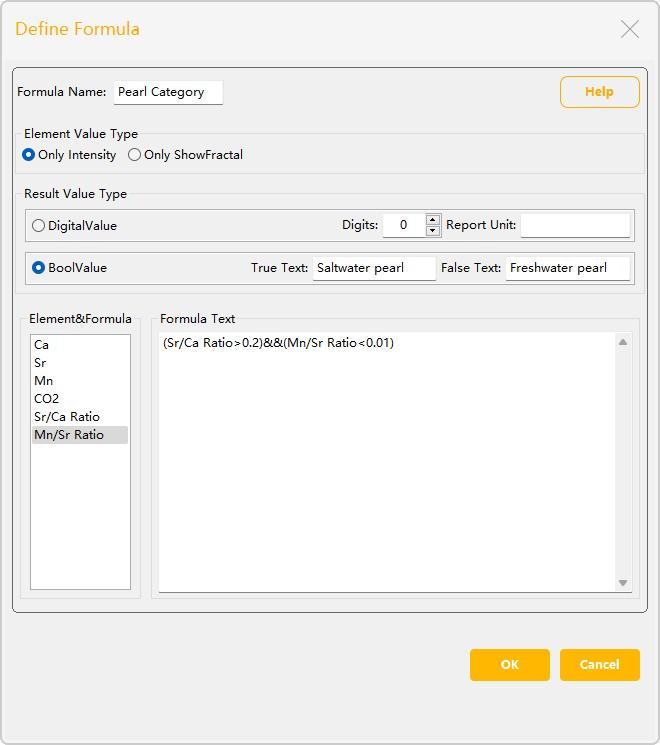f.Click on the "OK" button to add the current formula;

g.Once added, the list of formulas will show the name of the currently added formula, as shown below:

6.In the formula list screen, click the "OK" button to exit the Define Formula screen

7.In the Curves screen, click on the "Save" buttonto save the currently added formula, as shown below: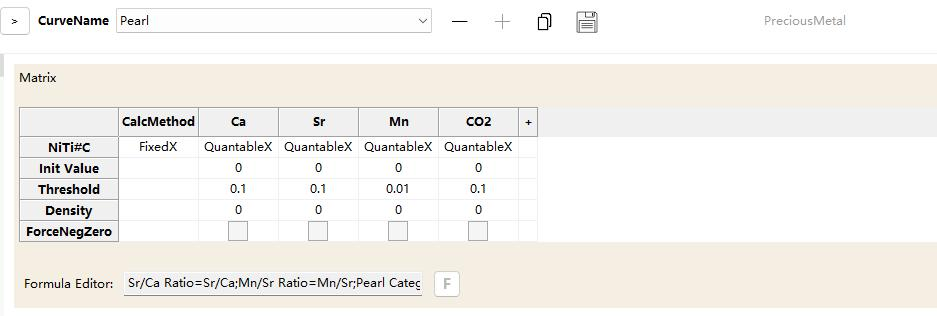8.Test sample validation formula, as shown below: